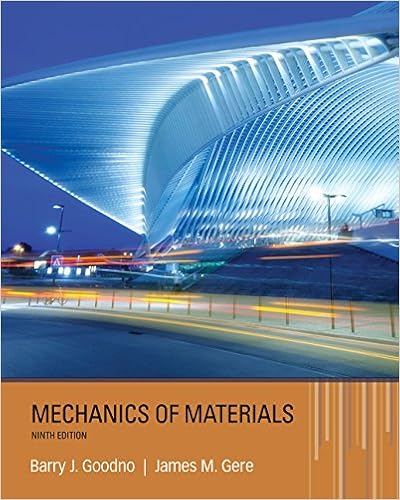# 5 2 9 4 13 6 10 216 9360 x x x x x x x x c since the

• Test Prep
• 16
• 100% (5) 5 out of 5 people found this document helpful

This preview shows page 8 - 11 out of 16 pages.

##### We have textbook solutions for you!
The document you are viewing contains questions related to this textbook.The document you are viewing contains questions related to this textbook.
Chapter 8 / Exercise 8.2-3
Mechanics of Materials
Gere/GoodnoExpert Verified
52!94!136!102169360xxxxxxxx. (c) Since the coefficient of x9is c9 = (9)(9)9(0)19!,(0)9!9!94!94!FFc= 5 6 7 8 = 1680.
##### We have textbook solutions for you!
The document you are viewing contains questions related to this textbook.The document you are viewing contains questions related to this textbook.
Chapter 8 / Exercise 8.2-3
Mechanics of Materials
Gere/GoodnoExpert Verified
MAT1322X Solution to Final Examination Summer 2018 9 Version B Part I. Multiple-choice Questions (2 12 = 24 marks) DBECFC ADDFEB 1. Which one of the following is closest tothe area of the region under the graph of y= 21xx, and above the line y= 2xin the first quadrant? (A) 0.11; (B) 0.09; (C) 0.13; (D) 0.10; (E) 0.12; (E) 0.08. Solution. (D) Let 21xx= 2x. Then x= 0 or x= 1. The area is 1112220001111111ln212122424xxxdxdxxdxduxxu0.09657. 2. Let Rbe the region in x-yplane above the parabola y= x2and under the line y= 2x. Let Sbe a solid obtained by revolving region Rabout the line y = −1. Then the volume of Scan be calculated by the integral (A) 42220((12 )(1) )xxdx; (B) 22220((12 )(1) )xxdx; (C) 222202((12 )(1) )xxdx; (D) 222202((12 )(1) )xxdx; (E) 42220((12 )(1) )xxdx; (F) 422202((12 )(1) )xxdx. Answer. (B) 3. Suppose a vertical cylindrical tank filled with oil of density kg/m3is buried underground so that the top is 3 meters under the ground surface. The radius of the tank is 6 meters, and the height of the tank is 10 meters. Let xbe the distance between a horizontal layer of oil and the top of the tank, and let gbe the acceleration of gravity. Then the work, in Joules, needed to pump out all the oil in the tank to the ground surface is calculated by the integral
MAT1322X Solution to Final Examination Summer 2018 10 Solution. (E) A layer of oil xmeters under the top of the tank with thickness dx has volume V(x) = 36dx. Its weight is w(x) = gV(x) = 36g dx. The work needed to pump this layer of oil to the ground is W(x) = w(x)(x+ 3) = 36g(x+ 3)dx. The total work needed is 10036(3)gxdx. 4. A triangular surface is submerged vertically into water with density kg/m3such that the top is 1 meter under the water surface as shown in the following figure. Let xbe the distance between a horizontal stripe of the surface and the water surface, and let gbe the gravity of acceleration, The force, in Newtons, acting on this surface can be calculated by the integral
•••